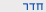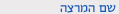שנה"ל תשע"והסתברות לתלמידי הנדסה ביו-רפואית
Probability for Biomedical engineering
0555-1140-01
הנדסה | תואר ראשון - הנדסה ביו-רפואיתסמ'  ב' 0800-1000 'ב 102 הנדסת תוכנה שיעור ד"ר אשכנזי גולן גלית
ש"ס:  3.0

סילבוס מקוצר
Objectives of the course: At the end of this course students should be able to (i) model problems in a probability-theory setting; (ii) solve probability problems; (iii) know the main distributions and probability concepts used in following statistic course .

Course description
 Contents 1 Basics of probability: Probability Space, Sets, Events 2 Combinatory: n! , n over k, Probabilities over a symmetric sample space 3 Conditional probability: Bayes' theorem, Dependent and independent events 4 Random variables: Definitions of Discrete and continuous random variables 5 Random variables (cont.): Expectation, Variance 6 Random variables (cont.): special random variables – binom, geometric, hyper-geometric,  Poisson (and Poisson process), exponential, Normal 7 Joint  Distributions: Joint Distributions, Independent variables 8 Joint  Distribution(cont): Conditional distributions, conditional expectation and variance Covariance, Pearson Correlation 9 Functions of several variables: Functions of several variables, sum of variables, expectation of sum of variables 10 Covariance: Variance of sum of variables, covariance, Pearson Correlation 11 More Random variables: bi-normal distribution, chi-square distribution, F-distribution 12 Central Limit Theorem: More on the Normal Distribution, , t-distribution 13 Distribution of Mean and Variance of a sampleהנדסה | תואר ראשון - הנדסה ביו-רפואית
0555-1140-01 הסתברות לתלמידי הנדסה ביו-רפואית
Probability for Biomedical engineering
שנה"ל תשע"ו | סמ'  ב' | ד"ר אשכנזי גולן גלית

סילבוס מפורט/דף מידע
- Homework assignments will be distributed in class on a weekly basis, with a due date of two weeks from the time of distribution.

- At the due date of each assignment, the corresponding solution will be distributed. The assignments will not be individually evaluated BUT submission will be recorded.
- Students are required to submit 80% of the total homework volume in order to be eligible to take the final exam. Homework should be submitted during class time.
- Grading percentages: 20% midterm, 80% final.

להצהרת הנגישות